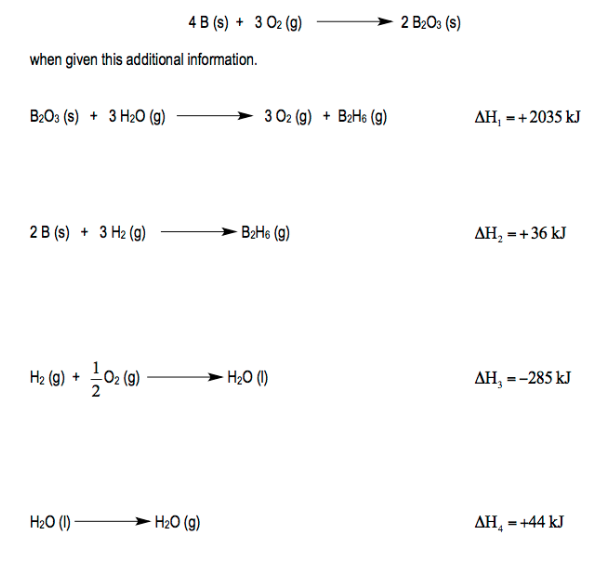# Problem: Calculate the enthalpy of the following reaction:

###### FREE Expert Solution
83% (328 ratings)
###### Problem Details

Calculate the enthalpy of the following reaction:What scientific concept do you need to know in order to solve this problem?

Our tutors have indicated that to solve this problem you will need to apply the Hess's Law concept. You can view video lessons to learn Hess's Law. Or if you need more Hess's Law practice, you can also practice Hess's Law practice problems.

What is the difficulty of this problem?

Our tutors rated the difficulty ofCalculate the enthalpy of the following reaction: ...as high difficulty.

How long does this problem take to solve?

Our expert Chemistry tutor, Jules took 8 minutes and 43 seconds to solve this problem. You can follow their steps in the video explanation above.

What professor is this problem relevant for?

Based on our data, we think this problem is relevant for Professor Bredas' class at GT.